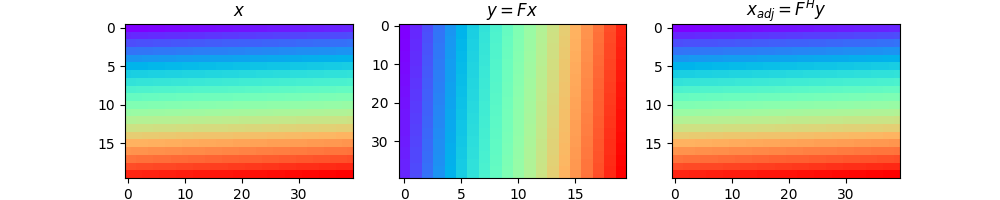# Transpose¶

This example shows how to use the pylops.Transpose operator. For arrays that are 2-dimensional in nature this operator simply transposes rows and columns. For multi-dimensional arrays, this operator can be used to permute dimensions

import matplotlib.pyplot as plt
import numpy as np

import pylops

plt.close("all")
np.random.seed(0)


Let’s start by creating a 2-dimensional array

dims = (20, 40)
x = np.arange(800).reshape(dims)


We use now the pylops.Transpose operator to swap the two dimensions. As you will see the adjoint of this operator brings the data back to its original model, or in other words the adjoint operator is equal in this case to the inverse operator.

Top = pylops.Transpose(dims=dims, axes=(1, 0))

y = Top * x.ravel()
y = y.reshape(Top.dimsd)

fig, axs = plt.subplots(1, 3, figsize=(10, 2))
fig.suptitle("Transpose for 2d data", fontsize=14, fontweight="bold", y=1.15)
axs.imshow(x, cmap="rainbow", vmin=0, vmax=800)
axs.set_title(r"$x$")
axs.axis("tight")
axs.imshow(y, cmap="rainbow", vmin=0, vmax=800)
axs.set_title(r"$y = F x$")
axs.axis("tight")
axs.set_title(r"$x_{adj} = F^H y$")
axs.axis("tight")Out:

(-0.5, 39.5, 19.5, -0.5)


A similar approach can of course be taken two swap multiple axes of multi-dimensional arrays for any number of dimensions.

Total running time of the script: ( 0 minutes 0.197 seconds)

Gallery generated by Sphinx-Gallery